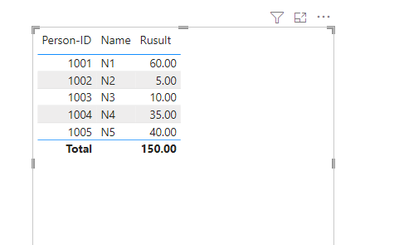cancel
Showing results for
Did you mean:Regular Visitor

## Finding related subtotals

I have this issue where I cannot find the related subtotals.

I have a datasource with these three tables, with simplified contents here:

Project-Sales

- Project-ID

- Invoice-value

Project-Member

- Project-ID

- Person-ID

Persons

- Person-ID

- Name

I have set up relations

Project-Member to Project-Sales: Many to One

Project-Member to Persons: Many to One

Now I want to summarize the sales subtotal for each person, but summarizing like

`CALCULATE(SUM(Project-Sales[Invoice-Value]))`

Only gives me the Total of all projects. How can I make the summary only use the subtotals for each person?

1 ACCEPTED SOLUTIONResolver I

As per your logic, I think it is simple to solve your problem per below measure,

Subtotal for Each =
sumx(
'Project-Member',
related('Project-Sales'[Invoice-value])
)
Let me know if it works.
4 REPLIES 4Community Support

Hi @JoaPalmer ,

You can try this method:

Sample data:In Project-Member table, new a measure:

``Count = CALCULATE(COUNT('Project-Member'[Project-ID]), ALLEXCEPT('Project-Member','Project-Member'[Project-ID]))``

Then new a table:

``Average = SUMMARIZE('Project-Member','Project-Member'[Project-ID],'Project-Sales'[Invoice-value], "Count", [Count])``

In Project-Member table, new a column:

``Per = CALCULATE(SUM('Average'[AVG]), FILTER('Average','Average'[Project-ID] = 'Project-Member'[Project-ID]))``

New a measure:

``Rusult = CALCULATE(SUM('Project-Member'[Per]), FILTER('Project-Member','Project-Member'[Person-ID]))``

The result is:Hope this helps you.

Here is my PBIX file.

Best Regards,

Community Support Team _Yinliw

If this post helps, then please consider Accept it as the solution to help the other members find it more quickly.Regular Visitor

Thank You for your extensive reply. However the result calculation you suggest is not correct.

In Your example, person 1001 is a member of project ID 1, 3 and 4. Sum of sales for those project is 10+30+40=80, not 60 as your calculation shows.

I don't know if there is an misunderstanding. I just want to get the summaries for the projects for each person involved, I don't understand why I would want to count rows or make average calculations?Resolver I

As per your logic, I think it is simple to solve your problem per below measure,

Subtotal for Each =
sumx(
'Project-Member',
related('Project-Sales'[Invoice-value])
)
Let me know if it works.Regular Visitor

This was so much easier solution, thank You very much, works perfect! 🙂